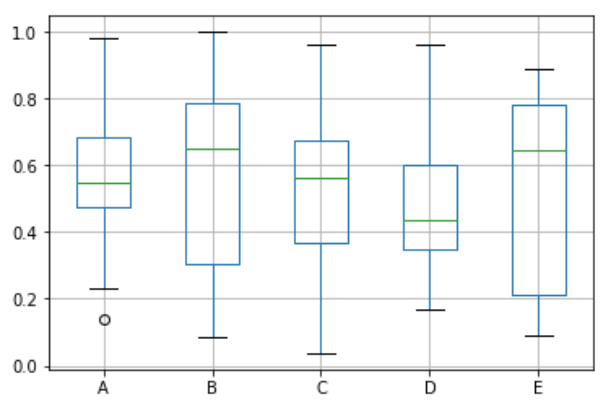# Python - Box Plots

Boxplots are a measure of how well distributed the data in a data set is. It divides the data set into three quartiles. This graph represents the minimum, maximum, median, first quartile and third quartile in the data set. It is also useful in comparing the distribution of data across data sets by drawing boxplots for each of them.

## Drawing a Box Plot

Boxplot can be drawn calling Series.box.plot() and DataFrame.box.plot(), or DataFrame.boxplot() to visualize the distribution of values within each column.

For instance, here is a boxplot representing five trials of 10 observations of a uniform random variable on [0,1).

```import pandas as pd
import numpy as np
df = pd.DataFrame(np.random.rand(10, 5), columns=['A', 'B', 'C', 'D', 'E'])
df.plot.box(grid='True')
```

Its output is as follows −## Useful Video Courses

Video

#### Python Online Training

187 Lectures 17.5 hours

Video

#### Python Essentials Online Training

55 Lectures 8 hours

Video

#### Learn Python Programming in 100 Easy Steps

136 Lectures 11 hours

Video

#### Python with Data Science

Best Seller

75 Lectures 13 hours

Video

#### Python 3 from scratch to become a developer in demand

Best Seller

70 Lectures 8.5 hours

Video

#### Python Data Science basics with Numpy, Pandas and Matplotlib

Most Popular

63 Lectures 6 hours